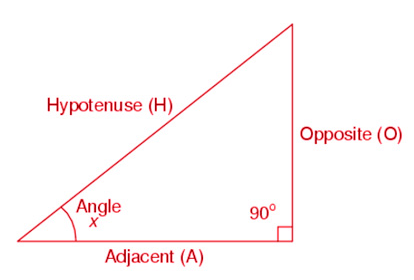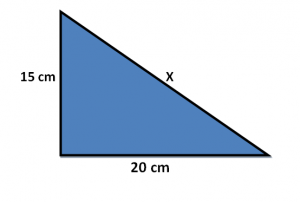# Right Angle Formula

Right angle is equal to 90 degrees. In a right angled triangle, the three sides are called: Perpendicular, Base(Adjacent) and Hypotenuse(Opposite). Perpendicular is the side that makes right angle with the base of the triangle.

The largest side side which is opposite to the right-angle(90 degree) is known as the Hypotenuse.  The side that is adjacent to the right angle are called legs cathetus. Thus both base and Perpendicular are known as Cathetus.

To find the side of the triangle, we need the sides of other two triangle.The Right angled triangle formula known as Pythagorean theorem (Pythagoras Theorem) is given by

$\large Hypotenuse^{2}=(Adjacent\;Side)^{2}+(Opposite\;Side)^{2}$

In trigonometry, the values of trigonometric functions at 90 degrees is given by:

Sin 90° = 1

Cos 90° = 0

Tan 90° = Not defined

Cot 90° = 0

Sec 90° = Not defined

Cosec 90° = 1

### Solved Examples

Question 1: Find is the value of X, where the 15 cm and 20 cm are the sides of the right-angled triangle?Solution:

Given:
$$\begin{array}{l}\sqrt{625}\end{array}$$